## 词向量：对word2vec的理解

2021/1/9 1:56:40 文章标签: word计算

## 三、Word2Vec

### 1、什么是word2vec？

word2vec的本质是一个神经网络语言模型，基于语言模型进行分布式词向量的训练。

### 2、CBOW模型和Skip-gram模型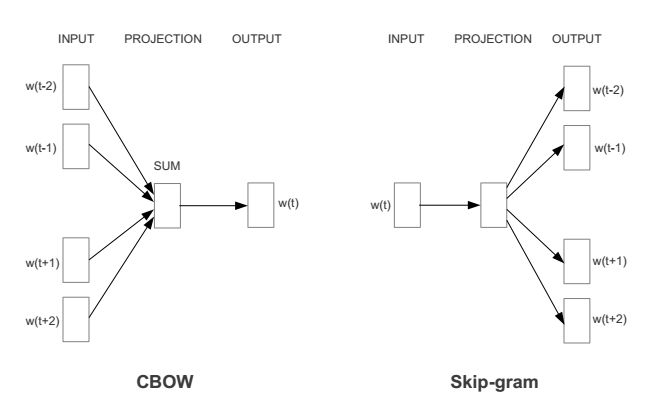#### （1）CBOW模型

CBOW模型是是在已知当前词的上下文的情况下，预测当前词#### （2）Skip-gram模型

Skip-gram模型则是在已知当前词的情况下，预测其上下文#### （3）模型其他知识

word2vec用了一种称为window的说法，实现了类似的上下文范围划定功能。

### 3、基于Hierarchical Softmax的CBOW模型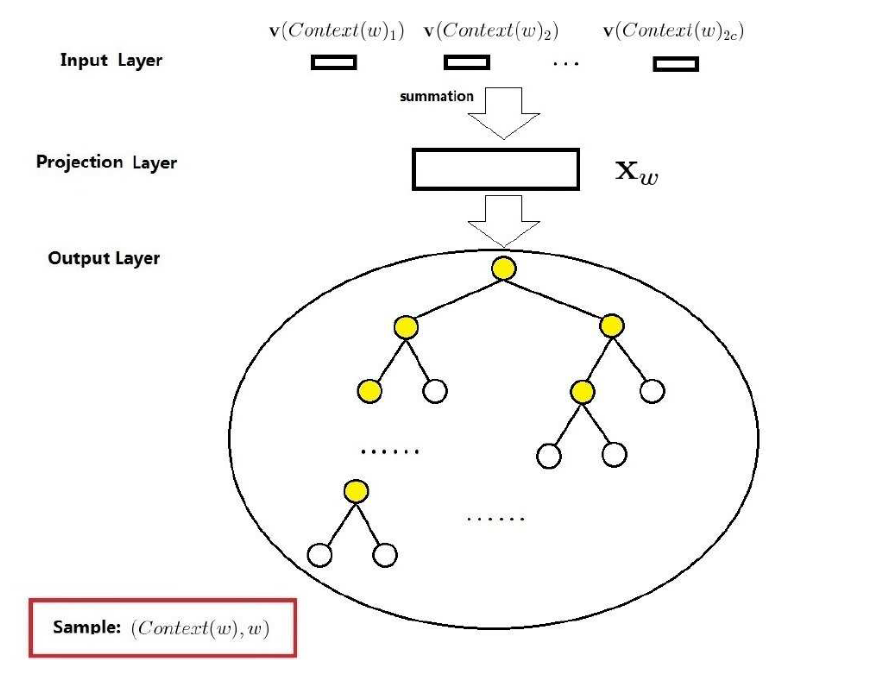* 输入层包含了中2c个词的词向量,...,

* 投影层将输入层的2c个向量做求和累加，合并成向量。

* 输出层对应一颗huffman二叉树，将V分类转换成多次二分类，得到输出。

#### （1）遍历语料，构造词典

``````for sentence_no, sentence in enumerate(sentences):
...
for word in sentence:
vocab[word] += 1``````

#### （2）对语料词典进行预处理

1）低频词的处理

``````for word, v in iteritems(self.raw_vocab):
if keep_vocab_item(word, v, min_count, trim_rule=trim_rule):
retain_words.append(word)
retain_total += v
``````

2）高频词的处理

``````for w in retain_words:
v = self.raw_vocab[w]
word_probability = (sqrt(v / threshold_count) + 1) * (threshold_count / v)``````

#### （3）构建huffman二叉树

1）构建huffman二叉树

``````heap = list(itervalues(self.wv.vocab))
heapq.heapify(heap)
for i in xrange(len(self.wv.vocab) - 1):
min1, min2 = heapq.heappop(heap), heapq.heappop(heap)
heapq.heappush(heap, Vocab(count=min1.count + min2.count, index=i + len(self.wv.vocab), left=min1, right=min2))``````

2）生成叶子节点对应的huffman编码

``````max_depth, stack = 0, [(heap, [], [])]
while stack:
node, codes, points = stack.pop()
if node.index < len(self.wv.vocab):
# leaf node => store its path from the root
node.code, node.point = codes, points
max_depth = max(len(codes), max_depth)
else:
# inner node => continue recursion
points = array(list(points) + [node.index - len(self.wv.vocab)], dtype=uint32)
stack.append((node.left, array(list(codes) + , dtype=uint8), points))
stack.append((node.right, array(list(codes) + , dtype=uint8), points))``````

#### （4）初始化模型参数

1）初始化叶子节点向量

``````self.wv.syn0 = empty((len(self.wv.vocab), self.vector_size), dtype=REAL)
# randomize weights vector by vector, rather than materializing a huge random matrix in RAM at once
for i in xrange(len(self.wv.vocab)):
# construct deterministic seed from word AND seed argument
self.wv.syn0[i] = self.seeded_vector(self.wv.index2word[i] + str(self.seed))``````

2）初始化非叶子节点向量

``self.syn1 = zeros((len(self.wv.vocab), self.layer1_size), dtype=REAL)``

#### （7）输出层处理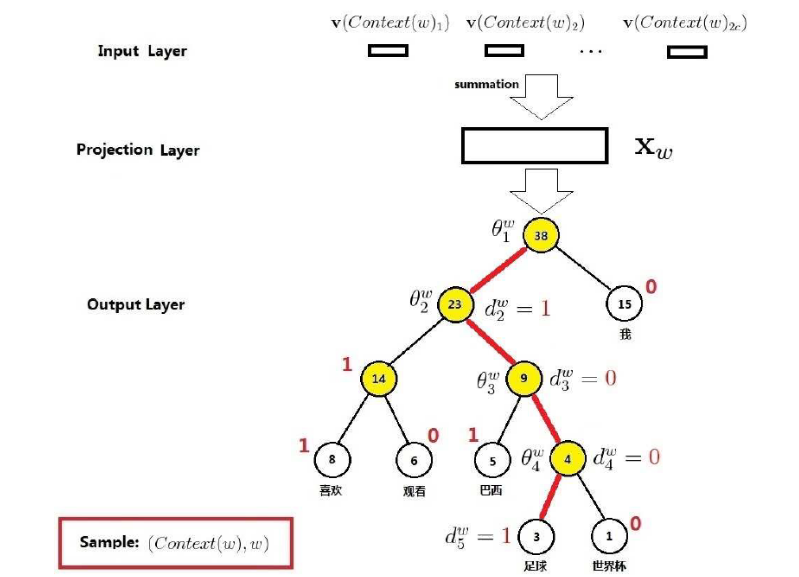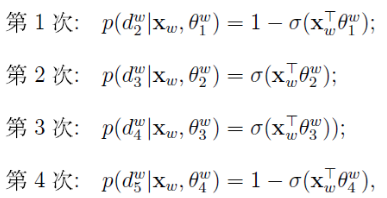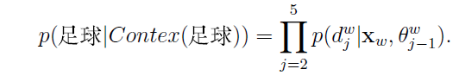#### （8）梯度优化

1）由上述条件概率公式，可以得到目标极大似然函数如下：

2）对上述表达式求求偏导，

3）那么，参数θ的更新公式如下：

4）词向量的更新公式如下：

``````        for sentence in sentences:
word_vocabs = [model.wv.vocab[w] for w in sentence if w in model.wv.vocab and
model.wv.vocab[w].sample_int > model.random.rand() * 2**32]  # 语料预检查，去除未在词典中出现及词频不满足要求的词
for pos, word in enumerate(word_vocabs):
reduced_window = model.random.randint(model.window)  # `b` in the original word2vec code
start = max(0, pos - model.window + reduced_window)
window_pos = enumerate(word_vocabs[start:(pos + model.window + 1 - reduced_window)], start)
word2_indices = [word2.index for pos2, word2 in window_pos if (word2 is not None and pos2 != pos)]
l1 = np_sum(model.wv.syn0[word2_indices], axis=0)  # 叶子节点向量
if word2_indices and model.cbow_mean:
l1 /= len(word2_indices)
train_cbow_pair(model, word, word2_indices, l1, alpha, compute_loss=compute_loss) 训练``````

``````        l2a = model.syn1[word.point]  # 中间节点向量
prod_term = dot(l1, l2a.T)
fa = expit(prod_term)  # propagate hidden -> output
ga = (1. - word.code - fa) * alpha  # vector of error gradients multiplied by the learning rate
if learn_hidden:
model.syn1[word.point] += outer(ga, l1)  # learn hidden -> output
neu1e += dot(ga, l2a)  # save error

# loss component corresponding to hierarchical softmax
if compute_loss: #  损失
sgn = (-1.0)**word.code  # ch function, 0-> 1, 1 -> -1
model.running_training_loss += sum(-log(expit(-sgn * prod_term)))``````

### 4、基于Hierarchical Softmax的Skip-gram模型

Skip-gram的基本计算方式与CBOW基本一致，不同的是skip-gram输入的是当前词的词向量，利用当前词向量预测上下文。

### 5、Negative Sampling模型

#### （2）怎么进行随机负采样？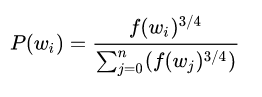## 四、参考

1、《数据结构》(c语言版)  严蔚敏等

2、《统计自然语言处理》  宗成庆

3、http://www.cnblogs.com/peghoty/p/3857839.html

4、Distributed Representations of Sentences and Documents  Mikolov

5、Efficient estimation of word representations in vector space  Mikolov

暂无相关的数据...